# Cube + square (second power, quadratic) - math problems

#### Number of problems found: 83

• Tower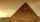Charles built a tower of cubes with an edge 2 cm long. In the lowest layer there were 6 cubes (in one row) in six rows, in each subsequent layer always 1 cube and one row less. What volume in cm3 did the whole tower have?
• Truncated cone 6Calculate the volume of the truncated cone whose bases consist of an inscribed circle and a circle circumscribed to the opposite sides of the cube with the edge length a=1.
• Wallpaper3750 cm square of wallpaper is needed to glue a cube-shaped box. Can Dad cut out the whole necessary piece of wallpaper as a whole if he has a roll of wallpaper 50 cm wide?
• TerezaThe cube has an area of base 256 mm2. Calculate the edge length, volume, and area of its surface.
• Cube wallsFind the volume and surface area of the cube if the area of one wall is 40cm2.
• Cube and sphereCube with the surface area 150 cm2 is described sphere. What is sphere surface?
• Cube diagonalsThe cube has a wall area of 81 cm square. Calculate the length of its edge, wall, and body diagonal.
• Wall diagonalCalculate the length of wall diagonal of the cube whose surface is 384 cm square.
• CubeCalculate the surface of the cube ABCDA'B'C'D' if the area of rectangle ACC'A' = 344 mm2.
• Rectangle poolFind dimensions of an open pool with a square bottom with a capacity of 32 m3 to have painted/bricked walls with the least amount of material.
• Cube 5The content area of one cube wall is 32 square centimeters. Determine the length of its edges, its surface and volume.
• PrismThree cubes are glued into a prism. The sum of the lengths of all its edges is 115 cm. What is the length of one edge of the original cube?
• CalculateCalculate the surface of the cube if its volume is 64 cm ^ 3.
• Cube diagonalsCalculate the length of the side and the diagonals of the cube with a volume of 27 cm3.
• The cube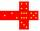The surface of the cube is 150 square centimeters. Calculate: a- the content of its walls b - the length of its edges c - its volume
• The body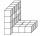The body on the figure consists of cubes with an edge length 10 cm. What surface has this body?
• Calculate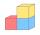Calculate the length of the wall diagonal of the cube with an edge 5 cm long.
• Grid/network of cube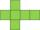The grid of the cube has a circumference of 42 mm. Calculate the surface area of the cube . ..
• Content area and percentsDetermine what percentage is smaller cube surface, when the surface area of the wall decreases by 25%.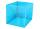I have homework. The cube has an edge 7 cm long and I must find wall and body diagonal.